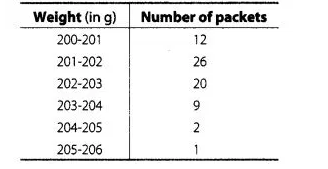# The weight of coffee in 70 packets are shown in the following table

Question:

The weight of coffee in 70 packets are shown in the following tableDetermine the model weight .

Solution:

In the given data, the highest frequency is 26, which lies in the interval 201-202

Here, $\quad l=201, f_{m}=26, f_{1}=12, f_{2}=20$ and (class width) $h=1$

$\because$ Mode $=l+\left(\frac{f_{m}-f_{1}}{2 f_{m}-f_{1}-f_{2}}\right) \times h=201+\left(\frac{26-12}{2 \times 26-12-20}\right) \times 1$

$=201+\left(\frac{14}{52-32}\right)=201+\frac{14}{20}=201+0.7=201.7 \mathrm{~g}$

Hence, the modal weight is 201.7 g.